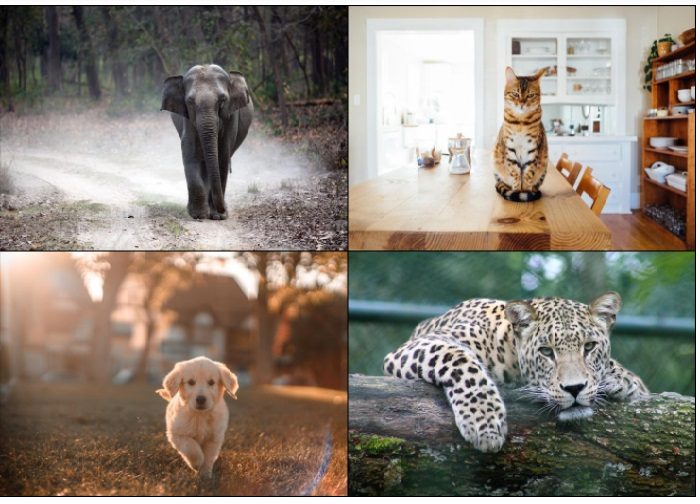# How to make a grid of images in PyTorch?

PyTorchServer Side ProgrammingProgramming

The torchvision.utils package provides us with the make_grid() function to create a grid of images. The images should be torch tensors. It accepts 4D mini-batch Tensor of shape (B ☓ C ☓ H ☓ W) or a list of tensor images, all of the same size.

• Here, B is batch size, C is the number of channels in the image, H and W are the height and width.

• H ☓ W of all images should be the same.

The output of this function is a torch tensor containing a grid of images. We can specify the number of images in a row using the nrow parameter. We have many other parameters to control the grid output. To visualize the grid of images, we first convert the whole grid to a PIL image.

## Syntax

torchvision.utils.make_grid(tensor)

## Parameters

• tensor - tensor or list of tensors. 4D mini-batch Tensor of shape (B x C x H x W) or a list of images all of the same size.

## Output

It returns a torch tensor containing a grid of images.

## Steps

• Import the required libraries. In all the following examples, the required Python libraries are torch and torchvision. Make sure you have already installed them.

import torch
import torchvision
from torchvision.utils import make_grid
• Read multiple JPEG or PNG images using the image_read() function. Specify the full image path with image types (.jpg or .png). The output of this function is a torch tensor of size [image_channels, image_height, image_width].

img1 = read_image('elephant.jpg')
img3 = read_image('dog.jpg')
• Make a grid of input images read as torch tensor using make_grid() function. Specify nrow to have number of images per row in the grid.

grid = make_grid([img1, img2, img3], nrow=3)

• Convert the grid tensor to a PIL image and display it.

img = torchvision.transforms.ToPILImage()(grid)
img.show()

## Example 1

In this Python program, we read three input images and make a grid of these images.

import torch
import torchvision
from torchvision.utils import make_grid

print("size of img1:", img1.size())
print("size of img2:", img2.size())
print("size of img3:", img3.size())

# make grid
grid = make_grid([img1, img2, img3])
print("size of grid:", grid.size())

# print("grid:\n", grid)
img = torchvision.transforms.ToPILImage()(grid)
img.show()

## Output

size of img1: torch.Size([3, 466, 700])
size of img2: torch.Size([3, 466, 700])
size of img3: torch.Size([3, 466, 700])
size of grid: torch.Size([3, 470, 2108])## Example 2

In the following Python program, we read four input images and make a grid of these images. We put nrow=2 to have two images in a row of the grid.

# Import the required library
import torch
import torchvision
from torchvision.utils import make_grid

print("Size of image:",img1.size())

# make grid
grid = make_grid([img1, img2, img3, img4], nrow = 2)
print("size of grid:", grid.size())

# print("grid:\n", grid)
img = torchvision.transforms.ToPILImage()(grid)
img.show()

## Output

Size of image: torch.Size([3, 466, 700])
size of grid: torch.Size([3, 938, 1406])